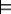### 5.4.2 Assumables and Conflicts

Reasoning from contradictions is a very useful tool. For many activities it is useful to know that some combination of assumptions is incompatible. For example, it is useful in planning to know that some combination of actions an agent is trying to do is impossible. It is useful in design to know that some combination of components cannot work together.

In a diagnostic application it is useful to be able to prove that some components working normally is inconsistent with the observations of the system. Consider a system that has a description of how it is supposed to work and some observations. If the system does not work according to its specification, a diagnostic agent must identify which components could be faulty.

To carry out these tasks it is useful to be able to make assumptions that can be proved to be false.

An assumable is an atom that can be assumed in a proof by contradiction. A proof by contradiction derives a disjunction of the negation of the assumables.

With a Horn clause knowledge base and explicit assumabes, if the system can prove a contradiction from some assumptions, it can extract combinations of assumptions that cannot all be true.

In a definite-clause knowledge base, a query is used to ask if a proposition is a consequence of the knowledge base. Given a query, the system tries to construct a proof for the query. With a proof by contradiction, the system tries to prove false. The user must specify what is allowable as part of an answer.

If KB is a set of Horn clauses, a conflict of KB is a set of assumables that, given KB, implies false. That is, C={c1,...,cr} is a conflict of KB if

KB∪{c1,...,cr}false.

In this case, an answer is

KB¬c1∨ ...∨ ¬cr.

A minimal conflict is a conflict such that no strict subset is also a conflict.

Example 5.19: In Example 5.17, if {c,d,e,f,g,h} is the set of assumables, then {c,d} and {c,e} are minimal conflicts of KB2; {c,d,e,h} is also a conflict, but not a minimal conflict.

In the examples that follow, the assumables are specified using the assumable keyword followed by one or more assumable atoms separated by commas.